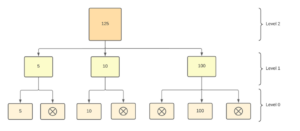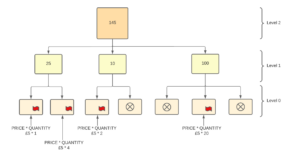Feeders are sometimes seen as a difficult issue within Planning Analytics (PA), but they don’t have to be. They solve a real modelling problem that we have in business reporting and planning systems, which in essence, answer the questions of how many or how much by ‘the who’, ‘the what’, ‘the where’ and ‘the when’ of an organisation.

PA is very efficient at calculating totals. It does not calculate every single cell. It only calculates cells that have values. This is important in a business solution because the number of cells rapidly increase. A simple cube of 4 dimensions with 2 years of 12 periods, a customer list of 100, a product list of 12, 4 geographical regions and 3 measures of price, quantity and revenue would have (2*12) * 100 * 12 * 4 * 3 data points or 172,800. This means there are lots of cells without any data in them.

Feeders are the way that PA squares the circle of sparsity and speed.

## How PA Calculates Totals

To get a value PA operates a ‘pull’ method. A total is only calculated when it is needed. It is not stored, awaiting use.

In the example below, PA calculates the ‘Level 2’ value of 125 by adding the 3 ‘Level 0’ values (5,10 and 100) when the user wants the value. It ignores the 4 cells with no value. Even in this simple example, over 60% of cells are ignored.This works when the values have been added to the cells.

## There’s more to life than totals

PA would be limited if it just let us add things up quickly. Business systems are all about relationships and conditions. When x, do Y. PA lets you add formulas to a cell. PA calls these formulas, rules.

Let’s assume that the ‘Level 0’ figure is the measure ‘Revenue’ which can be calculated by the formula or rule ‘Price’ * ‘Quantity’.

[‘Revenue’] = N: [‘Price] * [‘Quantity’];

PA does not store this calculated value of Revenue. This immense benefit is counteracted by the fact that PA now needs to check each ‘Revenue’ cell to see if there should be a calculated value there, and, as we have seen, there can be a lot of cells, most of which will have no values.

## We want our sparseness back

To get our speed back we must tell PA not to check all the ‘Revenue’ cells on the off chance that there is something there. We do this with the command ‘SKIPCHECK’.

That’s all well and good, but now PA will just see empty cells. It will be fast but will not have any values! This is where we must take responsibility and tell PA that there should be a value in this specific cell. This is where feeders come in. A feeder sets a flag which tells the database to evaluate the rule to get the value.To set this flag you would go to the section in the cube rules file after all the rules have been declared. This area is started with the command FEEDERS;